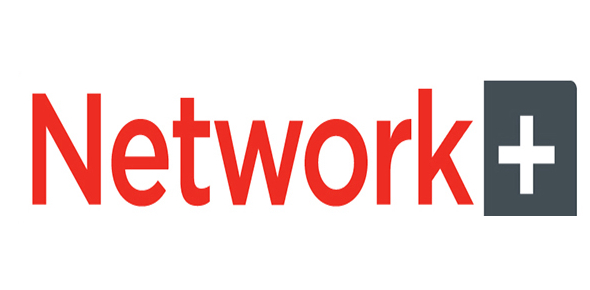# Network + Study Quiz (Chapter 3)

17 Questions | Total Attempts: 320SettingsThis is a quiz a composed from chapter 3 of Mike Meyer's network book.

Related Topics
• 1.
• A.

Bus

• B.

Ring

• C.

Star

• D.

Mesh

• 2.
Which of the following are Modern topologies? (select three)
• A.

Hybrid

• B.

Point-to-multipoint

• C.

Point-to-point

• D.

Star

• 3.
Which of the following describes a bus topology?
• A.

Connects via a single linear piece of cable

• B.

Connects via a central ring of cable

• C.

Offers fault tolerance

• D.

Uses a central connection

• 4.
True or false? With fault tolerance, if one cable breaks, all other computers could still communicate
• A.

True

• B.

False

• 5.
True or false? A segment is a bus shrunken into a box
• A.

True

• B.

False

• 6.
What is the difference between physical topology and signaling topology?
• A.

Physically describes how signals travel electronically, signaling describes how cables look

• B.

Physically describes how cables look, signaling describes how signals travel electronically

• 7.
Which two hybrid topologies were the only two to gain any popularity? (select two)
• A.

Star-ring

• B.

Ring-bus

• C.

Star-bus

• D.

Bus-ring

• 8.
What is the formula used to determine the number of connectors in a mesh topology
• A.

Y(y+1)/2

• B.

Y(y-1)/2

• C.

Y(y/1)2

• D.

2/y(y-1)

• 9.
Which is true about a Point-to-Multipoint topology?
• A.

A central device is not needed for members of a network to converse

• B.

A central device is needed for members of a network to converse

• C.

It is implemented in both wired and wireless networks

• D.

Two computers connect directly

• 10.
Which type of cable shields data transmissions from electronic magnetic interference (EMI)
• A.

Twisted-pair

• B.

Fiber-optic

• C.

Coaxial (coax)

• 11.
For early bus topologies, BNC connectors were most popular for which type of cable?
• A.

Coaxial

• B.

Twisted-pair

• C.

Fiber-optic

• 12.
CAT 5e cables are rated to handle 100mhz, but are capable of handling...?
• A.

10mbps

• B.

1000mbps

• C.

100mbps

• D.

10gbps

• 13.
The foru componenets of a fiber-optic cable are glass fiber (core), cladding, buffer material, and ______.
• 14.
Multimode cables use _______.
• 15.
Single-mode cables use _______.
• 16.
True or false? RS-232 is the recommended standard (RS) for serial communication
• A.

True

• B.

False

• 17.
Which fire rating provides no significant fire protection?
• A.

Plenum

• B.

Polyvinyl Chloride (PVC)

• C.

Riser